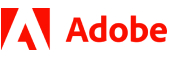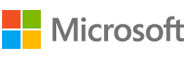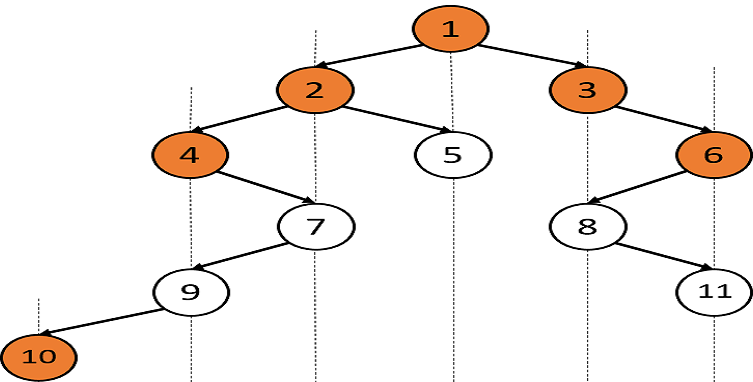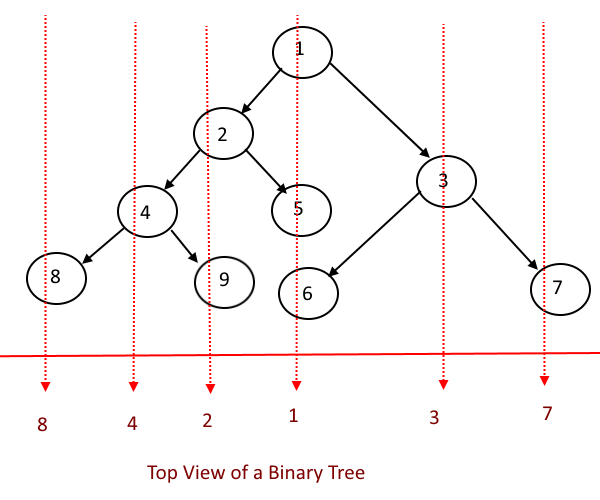New update is available. Click here to update.
Topics

# Top View Of Binary Tree

Moderate0/80
Average time to solve is 25m+25 more companies

## Problem statement

You are given a Binary Tree of 'n' nodes.

The Top view of the binary tree is the set of nodes visible when we see the tree from the top.

Find the top view of the given binary tree, from left to right.

Example :
``````Input: Let the binary tree be:
````````````Output: [10, 4, 2, 1, 3, 6]

Explanation: Consider the vertical lines in the figure. The top view contains the topmost node from each vertical line.
``````
Detailed explanation ( Input/output format, Notes, Images )
Sample Input 1:
``````1 2 3 4 5 -1 6 -1 7 -1 -1 8 -1 9 -1 -1 11 10 -1 -1 -1 -1 -1
``````

Sample Output 1:
``````10 4 2 1 3 6
``````

Explanation of Sample Output 1:
``````The binary tree is:
````````````Consider the vertical lines in the figure. The top view contains the topmost node from each vertical line.
``````

In test case 1,

Sample Input 2:
``````1 2 3 4 5 6 7 8 9 -1 -1 -1 -1 -1 -1 -1 -1 -1 -1
``````

Sample Output 2:
``````8 4 2 1 3 7
``````

Explanation of Sample Output 2:
``````The binary tree is:
````````````From left to right, the top view of the tree will be [8,4,2,1,3,7], where 9, 5 and 6 will be hidden when we see from the top of the tree.
``````

Expected time complexity :
``````The expected time complexity is O(n * log(n)).
``````

Constraints:
``````1 <= 'n' <= 10000
1 <= data in any node <= 10 ^ 6

Time limit: 1 sec
``````Console